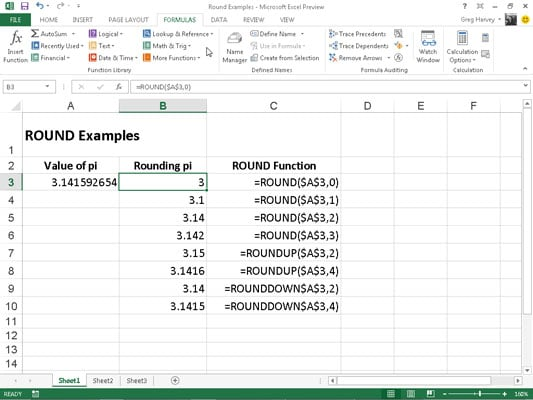##### Excel 2013 All-in-One For DummiesIn Excel 2013 you use the ROUND function found on the Math & Trig command button’s drop-down menu to round up or down fractional values in the worksheet as you might when working with financial spreadsheets that need to show monetary values only to the nearest dollar.

Unlike when applying a number format to a cell, which affects only the number’s display, the ROUND function actually changes the way Excel stores the number in the cell that contains the function. ROUND uses the following syntax:

`ROUND(number,num_digits)`

In this function, the number argument is the value that you want to round off, and num_digits is the number of digits to which you want the number rounded. If you enter 0 (zero) as the num_digits argument, Excel rounds the number to the nearest integer.

If you make the num_digits argument a positive value, Excel rounds the number to the specified number of decimal places. If you enter the num_digits argument as a negative number, Excel rounds the number to the left of the decimal point.

Instead of the ROUND function, you can use the ROUNDUP or ROUNDDOWN function. Both ROUNDUP and ROUNDDOWN take the same number and num_digits arguments as the ROUND function. The difference is that the ROUNDUP function always rounds up the value specified by the number argument, whereas the ROUNDDOWN function always rounds the value down.

The figure illustrates the use of the ROUND, ROUNDUP, and ROUNDDOWN functions in rounding off the value of the mathematical constant pi (π). In cell A3, the value of this constant is entered (with just nine places of nonrepeating fraction displayed when the column is widened) into this cell, using Excel’s PI function in the following formula:`=PI()`

Then the ROUND, ROUNDUP, and ROUNDDOWN functions in the cell range B3 through B10 were used to round this number up and down to various decimal places.

Cell B3, the first cell that uses one of the ROUND functions to round off the value of pi, rounds this value to 3 because you used 0 (zero) as the num_digits argument of its ROUND function (causing Excel to round the value to the nearest whole number).

Note the difference between using the ROUND and ROUNDUP functions both with 2 as their num_digits arguments in cells B5 and B7, respectively. In cell B5, Excel rounds the value of pi off to 3.14, whereas in cell B7, the program rounds its value up to 3.15.

Note that using the ROUNDDOWN function with 2 as its num_digits argument yields the same result, 3.14, as does using the ROUND function with 2 as its second argument.